# Quiz 4: Equivalence Calculations Under Inflation

Business

Rate of inflation: Average cost of a gallon gasoline was \$1.1 in 1996 which increased to \$4.12 in 15 years; hence, the rate of inflation can be calculated as follows:…… (1)Where, P = Present worth F = Future worth f = Rate of inflation N = Number of years Substitute the respective values in Equation (1) to obtain the rate of inflation.Hence, the rate of inflation is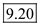percent. Price index in the year 2011: Price index can be calculated as follows:Price index in the year 2011 is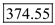.

Rate of inflation: Rate of inflation is 6 percent; hence, number of years required for a dollar (\$) to fall in its purchasing power to \$1.5 can be calculated as follows: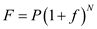…… (1)Where, P = Present worth F = Future worth f = Rate of inflation N = Number of years Time period: Rate of inflation is 6 percent; hence, number of years required for a dollar (\$) to fall in its purchasing power to one half can be calculated as follows:Hence, a dollar requires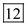years to fall its purchasing power to one half. Option (d) is correct.

Rate of inflation: Average cost of a gallon gasoline \$0.99 in 1998 increased to \$3.00 in 2011; hence, the rate of inflation can be calculated using the formula as below:…… (1)Where, P = Present worth F = Future worth f = Rate of inflation N = Number of years Substitute the respective values in Equation (1) to obtain the rate of inflation.Hence, the rate of inflation is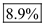.